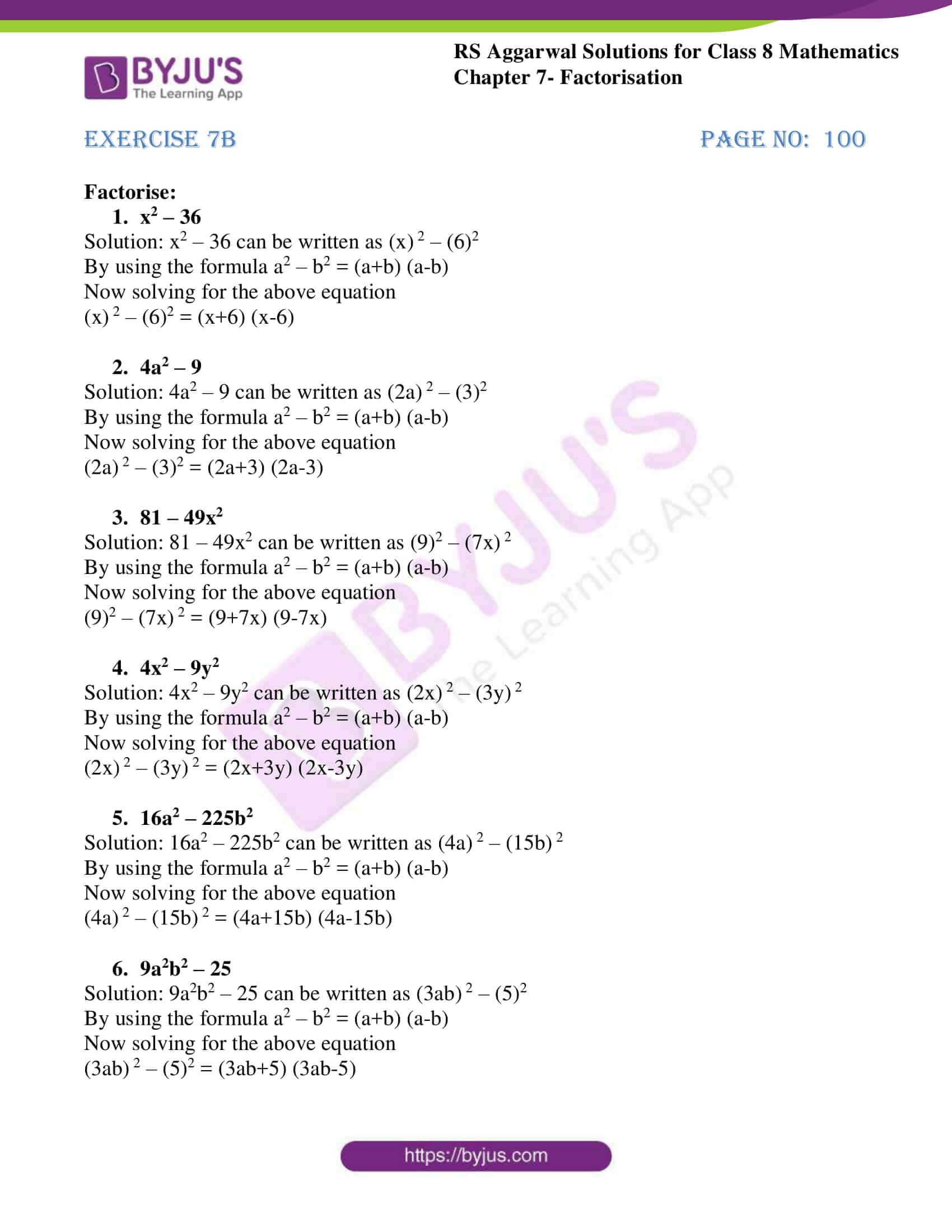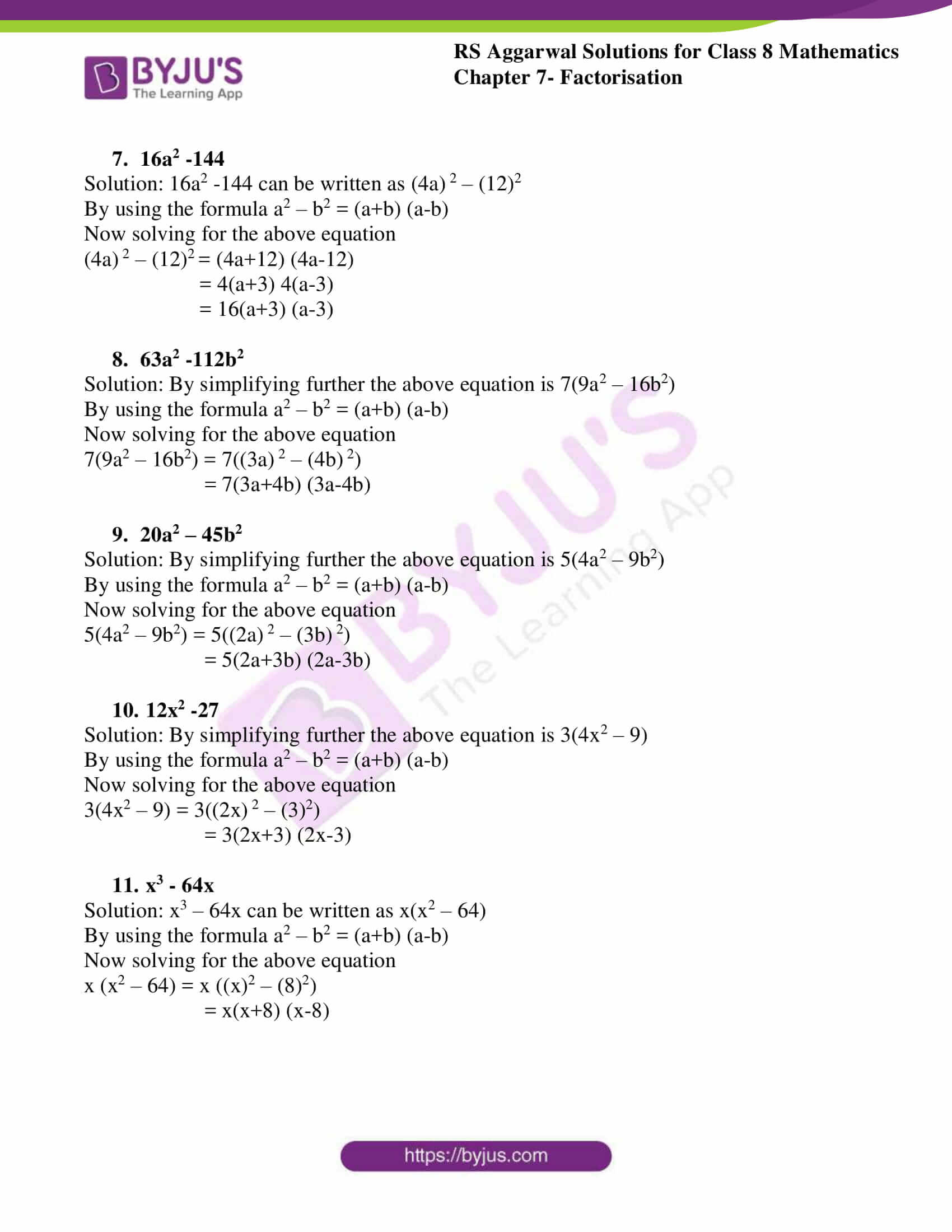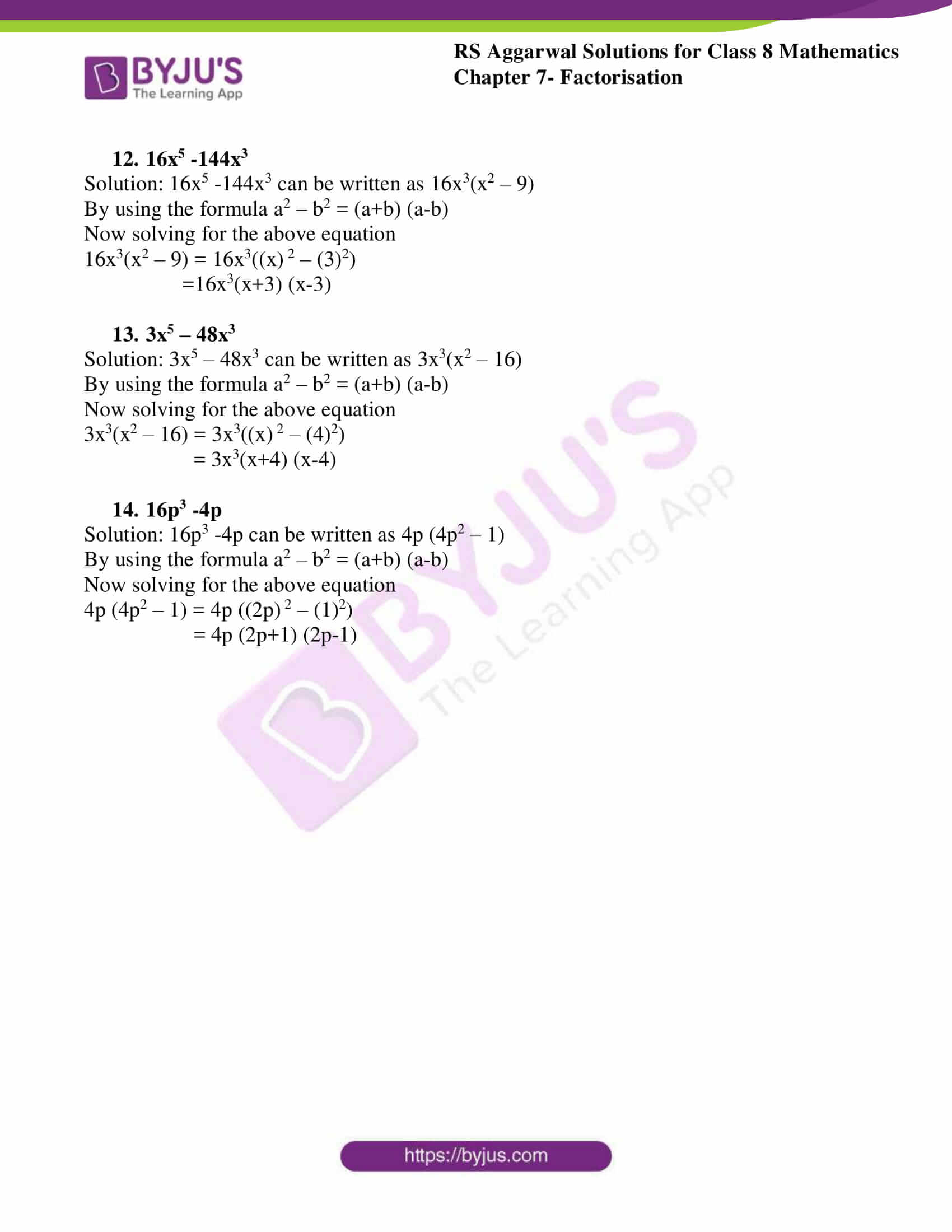# RS Aggarwal Solutions for Class 8 Chapter 7 - Factorisation Exercise 7B

Students can refer and download RS Aggarwal Solutions for Class 8 Maths Chapter 7- Exercise 7B, Factorisation from the links provided below. Our experts have solved the RS Aggarwal Solutions to ensure that the students are thorough with their basic concepts by practicing the solutions. In Exercise 7B of RS Aggarwal Class 8 Maths, we shall study one of the methods i.e. Factorisation when a given expression is the difference of two squares.

## Download PDF of RS Aggarwal Solutions for Class 8 Maths Chapter 7- Exercise 7B### Access Answers to RS Aggarwal Solutions for Class 8 Maths Chapter 7- Factorisation Exercise 7B

Factorise:

1. x2 – 36

Solution: x2 – 36 can be written as (x) 2 – (6)2

By using the formula a2 – b2 = (a+b) (a-b)

Now solving for the above equation

(x) 2 – (6)2 = (x+6) (x-6)

2. 4a2 – 9

Solution: 4a2 – 9 can be written as (2a) 2 – (3)2

By using the formula a2 – b2 = (a+b) (a-b)

Now solving for the above equation

(2a) 2 – (3)2 = (2a+3) (2a-3)

3. 81 – 49x2

Solution: 81 – 49x2 can be written as (9)2 – (7x) 2

By using the formula a2 – b2 = (a+b) (a-b)

Now solving for the above equation

(9)2 – (7x) 2 = (9+7x) (9-7x)

4. 4x2 – 9y2
5. Solution: 4x2 – 9y2 can be written as (2x) 2 – (3y) 2

By using the formula a2 – b2 = (a+b) (a-b)

Now solving for the above equation

(2x) 2 – (3y) 2 = (2x+3y) (2x-3y)

6. 16a2 – 225b2

Solution: 16a2 – 225b2 can be written as (4a) 2 – (15b) 2

By using the formula a2 – b2 = (a+b) (a-b)

Now solving for the above equation

(4a) 2 – (15b) 2 = (4a+15b) (4a-15b)

7. 9a2b2 – 25

Solution: 9a2b2 – 25 can be written as (3ab) 2 – (5)2

By using the formula a2 – b2 = (a+b) (a-b)

Now solving for the above equation

(3ab) 2 – (5)2 = (3ab+5) (3ab-5)

8. 16a2 -144

Solution: 16a2 -144 can be written as (4a) 2 – (12)2

By using the formula a2 – b2 = (a+b) (a-b)

Now solving for the above equation

(4a) 2 – (12)2 = (4a+12) (4a-12)

= 4(a+3) 4(a-3)

= 16(a+3) (a-3)

9. 63a2 -112b2

Solution: By simplifying further the above equation is 7(9a2 – 16b2)

By using the formula a2 – b2 = (a+b) (a-b)

Now solving for the above equation

7(9a2 – 16b2) = 7((3a) 2 – (4b) 2)

= 7(3a+4b) (3a-4b)

10. 20a2 – 45b2

Solution: By simplifying further the above equation is 5(4a2 – 9b2)

By using the formula a2 – b2 = (a+b) (a-b)

Now solving for the above equation

5(4a2 – 9b2) = 5((2a) 2 – (3b) 2)

= 5(2a+3b) (2a-3b)

11. 12x2 -27

Solution: By simplifying further the above equation is 3(4x2 – 9)

By using the formula a2 – b2 = (a+b) (a-b)

Now solving for the above equation

3(4x2 – 9) = 3((2x) 2 – (3)2)

= 3(2x+3) (2x-3)

12. x3 – 64x

Solution: x3 – 64x can be written as x(x2 – 64)

By using the formula a2 – b2 = (a+b) (a-b)

Now solving for the above equation

x (x2 – 64) = x ((x)2 – (8)2)

= x(x+8) (x-8)

13. 16x5 -144x3

Solution: 16x5 -144x3 can be written as 16x3(x2 – 9)

By using the formula a2 – b2 = (a+b) (a-b)

Now solving for the above equation

16x3(x2 – 9) = 16x3((x) 2 – (3)2)

=16x3(x+3) (x-3)

14. 3x5 – 48x3

Solution: 3x5 – 48x3 can be written as 3x3(x2 – 16)

By using the formula a2 – b2 = (a+b) (a-b)

Now solving for the above equation

3x3(x2 – 16) = 3x3((x) 2 – (4)2)

= 3x3(x+4) (x-4)

15. 16p3 -4p

Solution: 16p3 -4p can be written as 4p (4p2 – 1)

By using the formula a2 – b2 = (a+b) (a-b)

Now solving for the above equation

4p (4p2 – 1) = 4p ((2p) 2 – (1)2)

= 4p (2p+1) (2p-1)

## RS Aggarwal Solutions for Class 8 Maths Chapter 7- Exercise 7B

Exercise 7B of RS Aggarwal Class 8, Factorisation. This exercise mainly deals with Factorising when a given expression is the difference of two squares. The RS Aggarwal Solutions can help the students practice diligently while learning the fundamentals as it provides all the answers to the questions from the RS Aggarwal textbook. Students are suggested to practice the problems on a regular basis which will help them ace the exams and improve their overall percentage. Practicing as many times as possible helps in building time management skills and also boosts the confidence level to achieve high marks.